CONCAVITY AND CONVEXITY Analysis

• A function CONCAVE or concave down when the line joining any two points is found below the curve.

• Similarly, a function is CONVEX or concave up if the line joining any two points is found above the curve.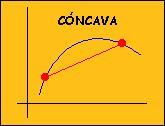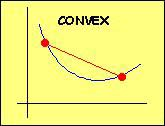• A POINT OF INFLEXION is a point where the graph of a function changes from being convex to concave or vice versa.

 1.Concavity and convexity 2.Finding points of inflexion 3.Applications

1. CONCAVITY AND CONVEXITY. POINTS OF INFLEXION

 A function y=f(x) is CONCAVE on an interval when the tangent to the curve at any point on this interval is above the curve.

 Change the value of x in the window and watch how the value of the derivative of the function decreases as x increases

• What is the sign of the second derivative over this interval?

A function y=f(x) is CONCAVE at x0 if it is in a neighbourhood of this point:

 If f''(xo)<0 then f is concave at xo

 Similarly, y=f(x) is CONVEX on an interval when the tangent to the curve at any point on this interval is below the curve.

 Watch how the derivative of the function changes in the window as the value of x is altered.
• In this case, what is the sign of the second derivative?

A function y=f(x) is CONVEX at x0 if it is in a neighbourhood of this point:

 If f''(xo)>0 then f is convex at xo

Now look carefully at the graphs of the function y=f(x) and its second derivative y=f''(x) in the window.

 Change the value of x in the window and check that f is concave when x<0 and convex when x>0
• What happens when x=0?

 When x=0 the curve changes the direction in which it is curving. It has a POINT of INFLEXION
• What is the behaviour of f''(0)?

• Note that the tangent to the curve crosses the curve at x=0

 If f''(xo)=0 and f changes the direction in which it is curving at xo then f has a point of inflexion at xo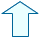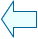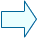María José García CebrianSpanish Ministry of Education. Year 2001